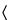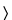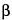696CRYSTALLINITY DETERMINATION BY SOLUTION CALORIMETRY

INTRODUCTION—THE CONCEPT OF CRYSTALLINITY
The perfectly ordered crystal lattice with every molecule in its expected lattice position is an ideal that is seldom, if ever, achieved. The other extreme is the amorphous state, in which a crystal contains the maximum possible density of imperfections (defects of various dimensionalities), such that all long-range order is lost while only the short-range order, imposed by its nearest molecular neighbors, remains. Real crystals lie somewhere between these two extremes. A crystal's position on a scale bounded by these two extremes is termed its crystallinity.
All real crystals, even in the pure state, possess some lattice imperfections or defects, which increase both the energy (enthalpy under conditions of constant atmospheric pressure) and the disorder (expressed as the entropy) of the crystal lattice. A crystal with a relatively small density of imperfections is said to be highly crystalline and to possess a high crystallinity. By contrast, a particle with a relatively high density of imperfections is said to be partially amorphous and to possess a low crystallinity. A totally amorphous particle corresponds to zero crystallinity. Even amorphous particles may contain domains of somewhat ordered molecules that can act as nuclei for crystallization; such so-called amorphous particles are said to possess a small but finite crystallinity.
For a powder or a collection of particles, two models of crystallinity have been proposed: the one-state model and the two-state model. According to the one-state model, all particles in the powder possess essentially the same crystallinity. By contrast, the two-state model postulates that each particle in a powder may be either crystalline or amorphous, such that the actual crystallinity is the weighted average of these two extreme crystallinities. In reality, a powder probably contains particles with different degrees of crystallinity, just as it may contain particles with different sizes and shapes. The lower the crystallinity of a particle, the greater its enthalpy and entropy. The increase in enthalpy is never totally compensated by the increase in entropy; however, the Gibbs free energy, which reflects the balance between them, actually increases. Hence, the lower the crystallinity of a particle, and consequently the greater its amorphous character, the greater its apparent intrinsic solubility, dissolution rate, and reactivity, but the lower its stability. Because of the great relevance of these properties, crystallinity is also an important property and requires measurement by a suitable method.
Various methods are available for the measurement of crystallinity. In the present chapter, the crystallinity of a powder is measured by solution calorimetry, although other methods could be used provided they have been validated.

DETERMINING CRYSTALLINITY BY SOLUTION CALORIMETRY
Solution calorimetry provides a means of determining enthalpy of solution (i.e., heat of solution under constant atmosphere pressure) of a solid substance. Enthalpy of solution may be defined as the enthalpy of the substance dissolved in the solution to a defined concentration minus the enthalpy of the original solid substance. The solvent for the solution process must be such that the weight of the solid taken (25 to 100 mg) dissolves within a time frame that matches the response time of the calorimeter, as discussed below. The enthalpy of solution is, of course, proportional to the amount of solid being dissolved. This amount may be defined as one mole for molar enthalpy or as one gram for specific enthalpy. If the substance is pure and if its molecular weight is known, the molar enthalpy is preferred, otherwise the specific enthalpy must be used. The enthalpy of solution is weakly dependent on both the temperature, which is usually 25.0, and the final concentration of the dissolved solute, which is usually on the order of 50 to 200 mg per 100 mL of solvent.
The crystallinity of the solid sample under study is given by the enthalpy of solution of the solid sample DHsx, minus the enthalpy of solution of the chosen reference standard of the same substance, DHsR, when determined under the same conditions. Because the reference standard is usually chosen for its perceived high crystallinity, its enthalpy of solution usually is algebraically greater (more endothermic or less exothermic) than that of the solid sample under study in the same solvent. Consequently, the crystallinity so determined is a negative quantity with the SI units, kJ/mol or J/g (J/kg is avoided because of its unwieldiness and potential for error). The preference for a negative value with respect to a highly crystalline reference standard recognizes the fact that most samples have a lower crystallinity than this reference standard.
A number of substances, including some purified by freeze-drying, may be available in an amorphous form but not in a crystalline form. With such substances, an amorphous form, prepared by a standard procedure, may be used as the reference standard. The enthalpy of solution may then be algebraically smaller than that of the chosen amorphous reference standard, in which case the crystallinity, as defined above, has a positive value.
The use of a single reference standard for each solid substance provides a single crystallinity scale, expressed in terms of energy, for each substance and recognizes that each solid drug or excipient has unique properties. Furthermore, the crystallinity can be recalculated—if the original reference standard is later replaced by a more crystalline (or more amorphous) reference standard—because enthalpies of solution are additive quantities according to Hess's Law of constant heat summation (i.e., the first law of thermodynamics).
In principle, the determination of the crystallinity of polymers may also be carried out using solution calorimetry, but this requires a defined reference standard for the polymer and a solvent in which the polymer is sufficiently soluble as discussed below.
Because the enthalpy of solution depends not only on the crystallinity of the solid but also on the various other solute–solute intermolecular interactions and on the solute–solvent and solvent–solvent intermolecular interactions, a zero value for the enthalpy of solution does not necessarily indicate zero crystallinity of the solid solute.
It is sometimes preferred to express the crystallinity, Pc , of a substance on a percentage scale, as described by Pikal et al.,* who also provide references to relevant earlier literature. This procedure requires two reference standards, namely a highly crystalline sample representing 100% crystallinity and having a measured enthalpy of solution of DHsc, and an essentially amorphous sample representing 0% crystallinity and having a measured enthalpy of solution of DHsa. From these values and from the measured enthalpy, DHss, of solution of the solid under study, the percentage crystallinity of the solid, Pc , may be calculated as follows:
Pc (%) = 100(DHssDHsa)/(DHscDHsa)
Clearly, crystallinity expressed on a percentage scale depends on three, not two, measured values and the enthalpies of solution may be replaced by other corresponding physical quantities that depend on crystallinity. The value of the percentage crystallinity of a solid sample, however, depends not only on the nature and method of preparation of the two reference standards, but also on the choice of the physical quantity that is measured.
The enthalpy of solution is measured at 25.0 ± 0.1either by an isoperibol (constant perimeter, i.e., jacket) solution calorimeter or by an isothermal (constant temperature) solution calorimeter using a fixed weight from 25 to 100 mg of solid sample, weighed to ±0.1 mg, with a fixed weight of solvent from 25 to 100 g, weighed to 0.01 g (usually 50.00 ± 0.01 g). The weight of solid solute and the nature of the solvent should be so chosen that the enthalpy of solution is not less than 200 mJ. At least three measurements are made with each sample, if a sufficient quantity is available, until the measured values of the heat of solution do not differ by more than 5%. The arithmetic mean of these three values is then calculated.
Isoperibol Solution Calorimetry
In the isoperibol solution calorimeter, the heat change during the solution process causes a corresponding change in temperature of the solvent–solute system (i.e., solution). This temperature change is measured by a temperature sensor, which is wired to an electrical circuit that records an electrical signal corresponding to the temperature change. Typically, this temperature change in an electronic form is measured at precisely defined time intervals to produce temperature–time data that are collected, analyzed by a computer, and then plotted. A blank run without addition of the solid solute to the solvent should show no discernible change in the slope of the temperature–time plot.
For isoperibol solution calorimeters, response is relatively rapid, but any heat losses to or heat gains from the bath reduce the accuracy and contribute to noise. Therefore, isoperibol solution calorimeters are more advantageous than isothermal solution calorimeters when the solution process is relatively fast. For all measurements of enthalpy of solution using isoperibol solution calorimeters, the choice of solvent and solid is critical. The nature and weight of the solvent and the weight of the solid sample allow the total heat change, corresponding to total dissolution of the solid, to proceed to completion within 10 minutes under vigorous stirring at a constant rotational speed within the range of 400 to 600 revolutions per minute. The rotational speed is checked with a stroboscope.
Isothermal Solution Calorimetry
In the isothermal (constant temperature) solution calorimeter, the heat change during the solution process is compensated for by an equal but opposite energy change, such that the temperature of the solvent–solute system (i.e., solution) remains constant. This equal but opposite energy change is measured and, when its sign is reversed, provides the enthalpy of solution. For isothermal calorimeters, response is relatively slow, but the compensation process eliminates the effects of heat losses to or heat gains from the bath. Therefore, isothermal calorimeters are more advantageous than isoperibol calorimetry when the solution process is relatively slow.

CALORIMETER CALIBRATION
To ensure the accuracy of the calorimeter, chemical calibrations must be performed daily. For an endothermic solution process, the calibration of the calorimeter is checked by measuring the heat absorbed during the dissolution of potassium chloride in distilled water at 298.15 K (25.0). The established enthalpy change in this endothermic process is 235.5 J/g or 4.196 kcal/mol. For an exothermic solution process, the calorimeter is checked by measuring the heat evolved during the dissolution of 5 g per L of tromethamine [tris(hydroxymethyl)aminomethane, THAM] in a 0.1 mol/L aqueous hydrochloric acid solution at 298.15 K (25.0). The established heat for the aforementioned process is –29.80 kJ/mol or –7.12 kcal/mol.
The effective heat capacity of the calorimeter cell and its contents is determined for every calorimeter run. This determination is accomplished by electrical heating of the contents of the calorimeter cell. The effective heat capacity is determined according to one of two protocols—either by making one determination after ampul breakage or by making one determination before and a second determination after ampul breakage and then averaging the two results. The accuracy and reliability of the electrical heating are established by the accuracy and reliability of the aforementioned chemical calibrations.

SAMPLE HANDLING
The thermodynamic stability of solids decreases with decreasing crystallinity. In particular, solids of low crystallinity, especially amorphous solids, tend to sorb water vapor from the atmosphere, leading to crystallization and a corresponding gain in crystallinity. For these reasons, anhydrous solid samples whose crystallinity is to be determined must be stored at zero humidity in sealed chambers containing a desiccant, preferably containing an indicator of effectiveness. If crystallinity–humidity studies are to be carried out, the solid sample should be stored in a sealed chamber containing a saturated salt solution to provide a defined relative humidity at 25.0 ± 0.1.

*  See Pikal, M.J.; Lukes, A.L.; Lang, J.E.; Gaines, K. Quantitative crystallinity determinations for-lactam antibiotics by solution calorimetry: correlations with stability, J. Pharm. Sci. 1978, 67, 767–773.

Auxiliary Information—